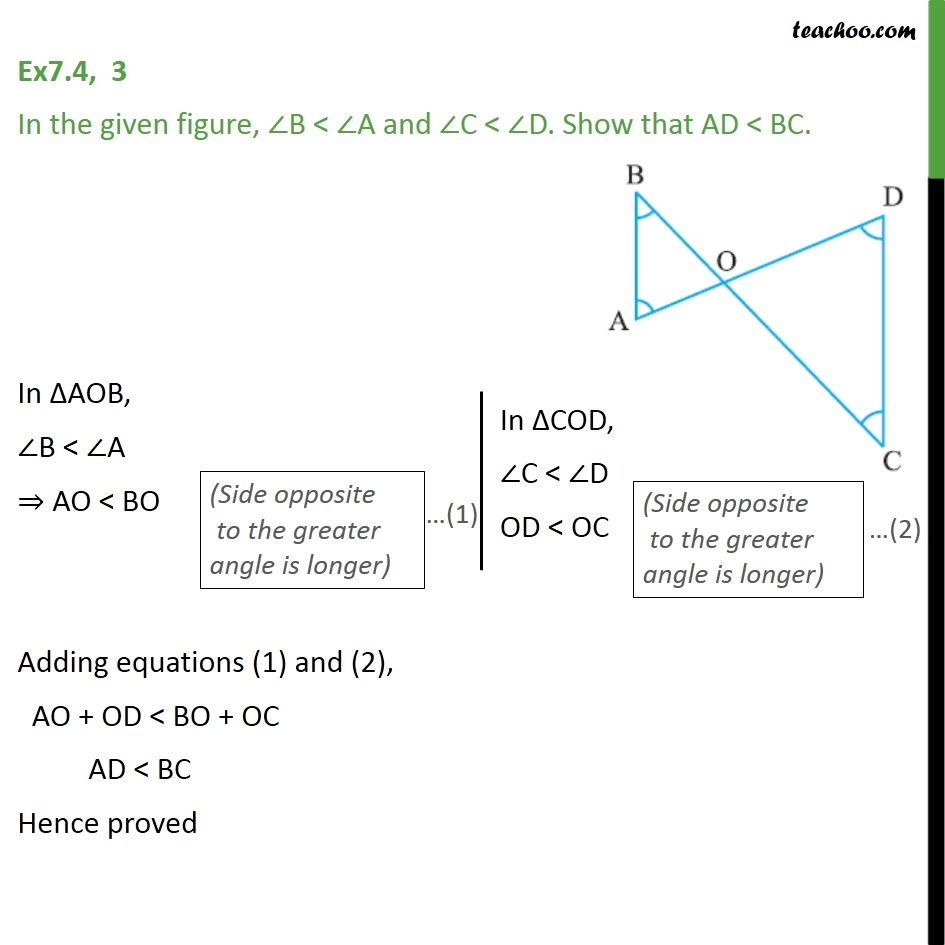1. Chapter 7 Class 9 Triangles
2. Serial order wise
3. Ex 7.4

Transcript

Ex7.4, 3 In the given figure, ∠B < ∠A and ∠C < ∠D. Show that AD < BC. In ΔAOB, ∠B < ∠A ⇒ AO < BO (Side opposite to the greater angle is longer) In ΔCOD, ∠C < ∠D OD < OC (Side opposite to the greater angle is longer) Adding equations (1) and (2), AO + OD < BO + OC AD < BC Hence proved

Ex 7.4

Chapter 7 Class 9 Triangles
Serial order wise